# Using Run Charts to Visualise Variant CPs Due to Changing Targets of A&E Waiting Time Data

### February 27, 2014 | Alan

In analytics, run charts are used to identify and display trends in data over time. We will show how we can visualise variant CPs due to changing targets of A&E waiting time data using run charts created with ggplot2 where the CP (centre point) is the median of the data. This enables us to look at multiple timeframes within our data that correspond to each target. We can then plot the median and the upper and lower limits for every defined target. We then embed this code into a function.

### Output

In this tutorial we will learn how to create a run chart similar to the following: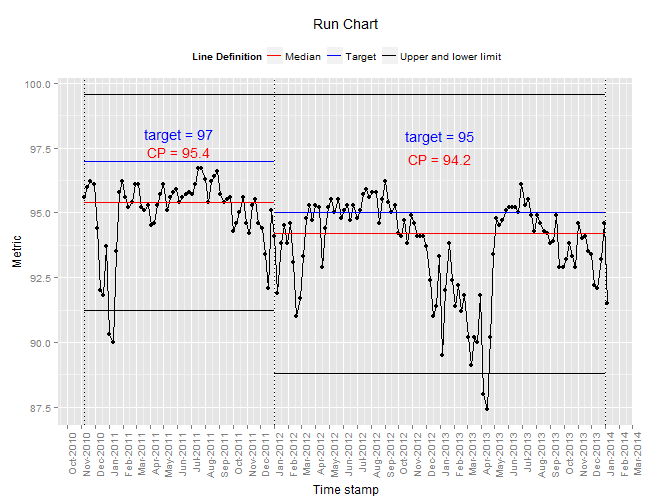### Related Blog Posts

This post is one of a series that is intended to be read in sequence. These posts are:

We suggest you initially read the blog posts in this order to help you understand the worked examples.

Other related blogs are:

### Data Source

This dataset is called `ae_england_20140501` and is available in the Open Data workspace. This is an open dataset that has been sourced from here, cleaned up and converted to a CSV file and loaded into the AnalytiXagility platform.

### Function Name

We will write the code to visualise variant CPs on run charts, and then show how to wrap this up into a function: `run_selected_targeting()`.

### Learning Outcomes

We focus mainly on the `ggplot2` package in this post. We consider the construction of a layered plot, formatting labels and annotation, as well as formatting the legend. We also demonstrate some of the techniques used when writing functions.

## Workflow

### Step 1 – Set up the environment

Load the relevant libraries:

``````library(ggplot2)
library(scales)
``````

Import data into the R console:

``````ae_data <- xap.read_table("ae_england_20140105")
``````

Define the date and metric variables. To do this, use the `as.Date()` function to represent `ae_data\$period` in date format:

``````run_date <- as.Date(ae_data\$period, format = "%Y-%m-%d")
run_metric <- ae_data\$percentagein4hoursorless_type1
``````

`ggplot()` requires data to be in a dataframe for plotting purposes, so we save this data as a dataframe and name the columns accordingly:

``````to_plot <- data.frame(run_date, run_metric)
colnames(to_plot) <- c("run_date", "run_metric")
``````

### Step 2 – Define and calculate parameters

In our blog Visualising features of A&E waiting time data using run charts we calculated the median and upper and lower limits for the entire dataset based on one target spanning the data. Now let us consider varying the targets in our data.

Let’s say the target for the percentage of people being seen within 4 hours is 97% between the start of 2010 and the end of 2011. This is then reduced to 95% for the remaining time. We need to calculate these parameters for each target. First we need to specify the targets and the corresponding timeframes. The data spans the following dates:

``````min(to_plot\$run_date)
``````
``````##  "2010-11-07"
``````
``````max(to_plot\$run_date)
``````
``````##  "2014-01-05"
``````

Define the targets and timeframes as vectors:

``````timeframes <- as.Date(c("2010-11-07", "2011-12-31", "2014-01-05"))
targets <- c(97, 95)
``````

The timeframes vector defines the break points between time periods where targets have changed. For every target we need to calculate the corresponding CP (centre point). We need to define the first and last date in our data, and the point where the target changes so we have two time periods:

• 2010-11-07 to 2011-12-31
• 2011-12-31 to 2014-01-05.

We can now calculate the parameters for the first time interval: 2010-11-07 to 2011-12-31.

Firstly calculate the CP (centre point):

``````cp1 <- median(to_plot\$run_metric[(to_plot\$run_date) >= timeframes & (to_plot\$run_date) <=
timeframes])
``````

Compute the upper and lower limits for this interval:

``````std_dev1 <- sd(to_plot\$run_metric[(to_plot\$run_date) >= timeframes & (to_plot\$run_date) <=
timeframes], na.rm = TRUE)
three_sd1 <- 3 * std_dev1
ul1 <- cp1 + three_sd1
ll1 <- cp1 - three_sd1
``````

Let’s do the same for the second time interval: 2011-12-31 to 2014-01-05:

``````cp2 <- median(to_plot\$run_metric[(to_plot\$run_date) >= timeframes & (to_plot\$run_date) <=
timeframes])
std_dev2 <- sd(to_plot\$run_metric[(to_plot\$run_date) >= timeframes & (to_plot\$run_date) <=
timeframes], na.rm = TRUE)
three_sd2 <- 3 * std_dev2
ul2 <- cp2 + three_sd2
ll2 <- cp2 - three_sd2
``````

### Step 3 – Start plotting

Now we can build our plot! We will begin by constructing a basic plot that we can add layers to. More details on how this initial plot is created can be found in other blogs:

We need:

``````m <- ggplot(colour = "Legend") + geom_line(data = to_plot, aes(x = run_date,
y = run_metric)) + geom_point(data = to_plot, aes(x = run_date, y = run_metric)) +
ggtitle("Run Chart") + xlab("Time stamp") + ylab("Metric") + scale_x_date(breaks = date_breaks("months"),
labels = date_format("%b-%Y")) + theme(axis.text.x = element_text(angle = 90,
hjust = 1), legend.position = "top")
m
``````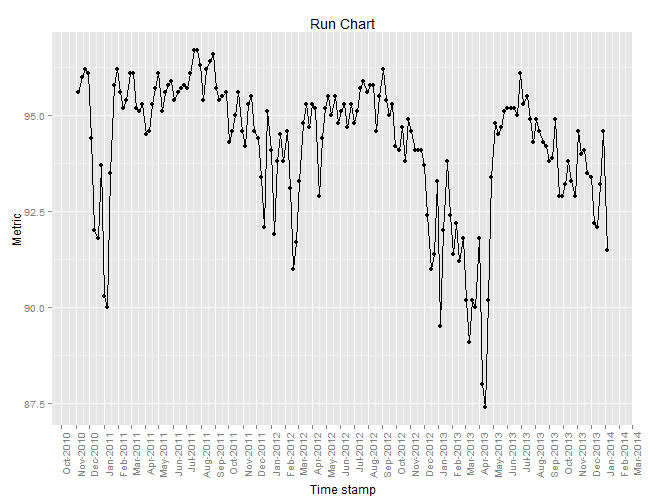Let’s start adding some layers to this. First we define the x-axis positions of the start and end points of the line segments we will be plotting:

``````xstart <- timeframes[1:2]
xfinish <- timeframes[2:3]
``````

Next we add the centre points to the plot using `geom_segment()` setting the x and y coordinates of the line segments:

``````n <- m + geom_segment(aes(x = xstart, y = c(cp1, cp2), xend = xfinish, yend = c(cp1,
cp2), colour = "Median  "))
n
``````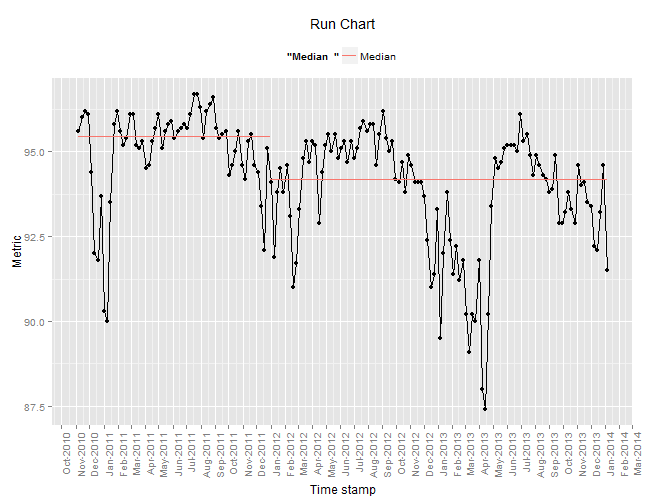Add the upper and lower limits, and the targets:

``````o <- n + geom_segment(aes(x = xstart, y = c(ul1, ul2), xend = xfinish, yend = c(ul1,
ul2), colour = "Upper and lower limit ")) + geom_segment(aes(x = xstart,
y = c(ll1, ll2), xend = xfinish, yend = c(ll1, ll2), colour = "Upper and lower limit ")) +
geom_segment(aes(x = xstart, y = targets, xend = xfinish, yend = targets,
colour = "Target  "))

o
``````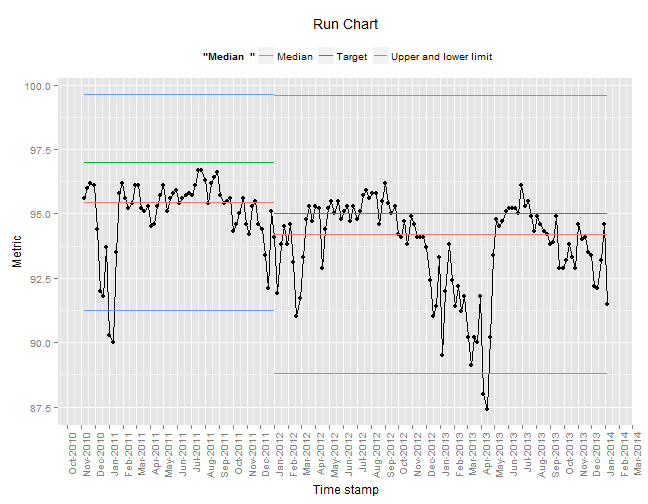That’s all the parameters, now we need to make it look a bit better! Firstly, let’s use `geom_vline()` to add some vertical lines that indicate the different time intervals more clearly:

``````p <- o + geom_vline(aes(xintercept = as.numeric(timeframes)), linetype = "dotted")
p
``````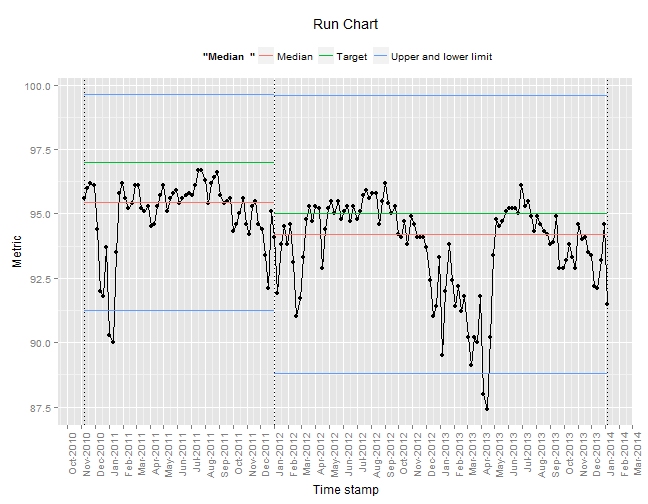We can also change the default colours of the lines using `scale_colour_manual()` and add a relevant title to the legend. More information can be found here.

``````q <- p + scale_colour_manual(values = c(`Median  ` = "red", `Target  ` = "blue",
`Upper and lower limit ` = "black"), guide = guide_legend(title = "Line Definition"))
q
``````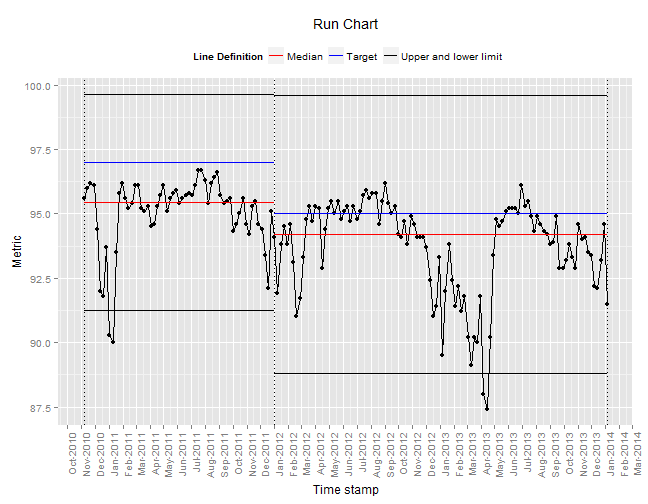### Step 4 – Annotate

We can add annotations to our plot using `annotate()`. For this example it would be useful to display the value of the CP and the target for each time interval. We need to supply the x and y coordinates of the annotations and the text for it to display:

``````median_label1 <- paste("CP = ", cp1, sep = "")
median_label2 <- paste("CP = ", cp2, sep = "")
target_label1 <- paste("target = ", targets, sep = "")
target_label2 <- paste("target = ", targets, sep = "")

xposition1 <- timeframes + (timeframes - timeframes)/2
xposition2 <- timeframes + (timeframes - timeframes)/2
ymed1 <- ul1 - 1
ymed2 <- ul2 - 1
ytarget1 <- ul1 - 2
ytarget2 <- ul2 - 2
``````

And then add them to the plot:

``````r <- q + annotate("text", x = c(xposition1, xposition2), y = c(ymed1, ymed2),
label = c(median_label1, median_label2), colour = "red") + annotate("text",
x = c(xposition1, xposition2), y = c(ytarget1, ytarget2), label = c(target_label1,
target_label2), colour = "blue")
r
``````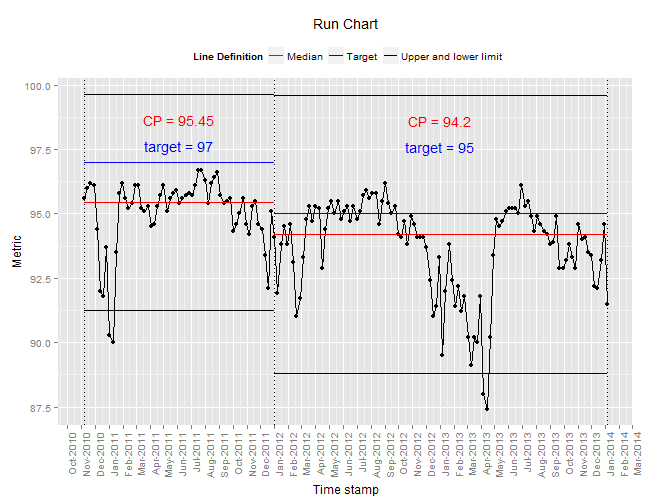### Step 5 – Steps 2-4 in a function

Writing the above code into a function allows us to reuse it efficiently for other datasets. The function has the parameters:

• `timeframes` (start and end points of the time intervals for the corresponding targets)
• `targets` (target values)
• `dataset` (name of the dataset to plot)
• `date_var` (name of the date variable column in the dataset)
• `metric_var` (name of the metric variable column in the dataset).

The function loops round each target and the corresponding time interval and calculates:

• The CP.
• The upper and lower limits.
• The positions of the annotations.
The method for how each of these is calculated is the same as above, however in this function we calculate the positions of the annotations depending on the scale of the dataset. The function then returns the run chart with the varying targets.

It is also important to note that global variables are assigned in this function because they are required for the final plot that is returned. To assign a global variable we write `variable<<-1+1` rather than `variable<-1+1`.

``````run_selected_targeting <- function(timeframes, targets, dataset, date_var, metric_var) {
library(ggplot2)
xstart <<- c(timeframes[1:(length(timeframes) - 1)])
xfinish <<- c(timeframes[2:(length(timeframes))])
cpf <<- numeric()
ulf <<- numeric()
llf <<- numeric()
median_labels <<- character()
target_labels <<- character()
x_label_positions <<- as.Date("1900-01-01", "1900-01-01")
y_cp_label_positions <<- numeric()
y_target_label_positions <<- numeric()

mm <- ggplot(colour = "Legend") + geom_line(data = dataset, aes_string(x = date_var,
y = metric_var)) + geom_point(data = dataset, aes_string(x = date_var,
y = metric_var)) + ggtitle("Run Chart") + xlab("Time stamp") + ylab("Metric") +
scale_x_date(breaks = date_breaks("months"), labels = date_format("%b-%Y")) +
theme(axis.text.x = element_text(angle = 90, hjust = 1), legend.position = "top")

for (i in 1:length(targets)) {
cpf[i] <<- median(dataset[, metric_var][(dataset[, date_var]) >= timeframes[i] &
(dataset[, date_var]) <= timeframes[i + 1]])
std_dev <- sd(dataset[, metric_var][(dataset[, date_var]) >= timeframes[i] &
(dataset[, date_var]) <= timeframes[i + 1]], na.rm = TRUE)
three_sd <- 3 * std_dev
ulf[i] <<- cpf[i] + three_sd
llf[i] <<- cpf[i] - three_sd
median_labels[i] <<- paste("CP = ", cpf[i], sep = "")
target_labels[i] <<- paste("target = ", targets[i], sep = "")
x_label_positions[i] <<- timeframes[i] + (timeframes[i + 1] - timeframes[i])/2
maxpos <- max(dataset[, metric_var][dataset[, date_var] >= timeframes[i] &
dataset[, date_var] <= timeframes[i + 1]])
minpos <- min(dataset[, metric_var][dataset[, date_var] >= timeframes[i] &
dataset[, date_var] <= timeframes[i + 1]])
y_cp_label_positions[i] <<- maxpos + ((maxpos - minpos)/10)
y_target_label_positions[i] <<- maxpos + 2 * ((maxpos - minpos)/10)
}

run <- mm + geom_segment(aes(x = xstart, y = targets, xend = xfinish, yend = targets,
colour = "Target  ")) + geom_segment(aes(x = xstart, y = cpf, xend = xfinish,
yend = cpf, colour = "Median  ")) + geom_segment(aes(x = xstart, y = ulf,
xend = xfinish, yend = ulf, colour = "Upper and lower limit  ")) + geom_segment(aes(x = xstart,
y = llf, xend = xfinish, yend = llf, colour = "Upper and lower limit  ")) +
geom_vline(aes(xintercept = as.numeric(timeframes)), linetype = "dotted") +
scale_colour_manual(values = c(`Median  ` = "red", `Target  ` = "blue",
`Upper and lower limit  ` = "black"), guide = guide_legend(title = "Line Definition")) +
annotate("text", x = c(x_label_positions), y = c(y_cp_label_positions),
label = c(median_labels), colour = "red") + annotate("text", x = x_label_positions,
y = y_target_label_positions, label = c(target_labels), colour = "blue")
return(run)
}

run_selected_targeting(timeframes, targets, dataset = to_plot, date_var = "run_date",
metric_var = "run_metric")
``````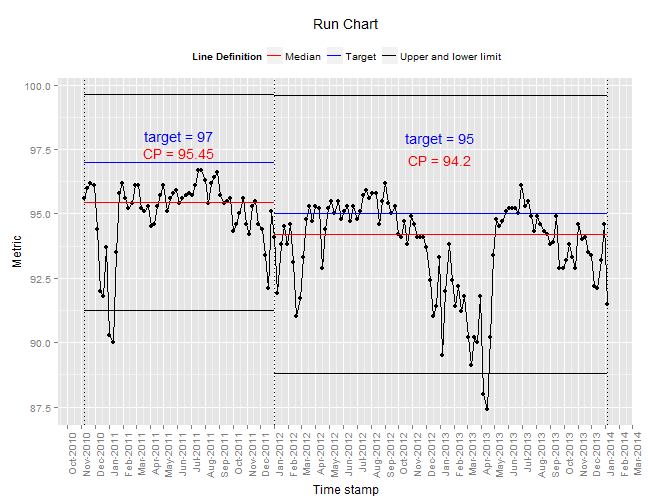Let’s now say the targets are:

• 97% from 2010 to the end of 2011
• 94% for 2012
• 95% thereafter.

We can plot this easily by altering the two parameters, `targets` and `timescales`:

``````timeframes <- as.Date(c("2010-11-07", "2011-12-31", "2012-12-31", "2014-01-05"))
targets <- c(97, 94, 95)
``````

And then run the function:

``````run_selected_targeting(timeframes, targets, dataset = to_plot, date_var = "run_date",
metric_var = "run_metric")
``````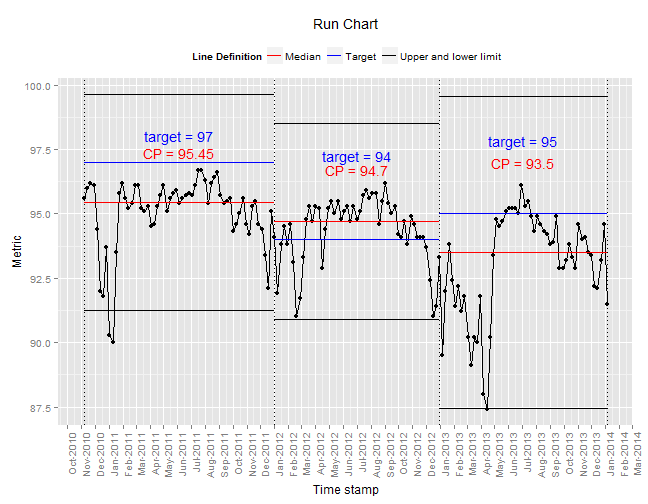#### admin

This site uses Akismet to reduce spam. Learn how your comment data is processed.